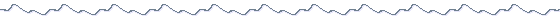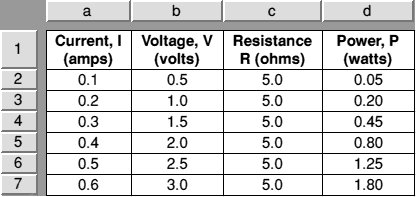Spreadsheets are very useful tools for scientists. They allow us to enter data from experiments very easily into neat and tidy tables, (the standard way of presenting your results), allow complex manipulation of the results, and will even create graphs. As an example, we may have measured voltage (V) and current (I) in an experiment. The data can be put into a spreadsheet, and the software can be told to combine the two to find RESISTANCE (R), or POWER (P). The relationships:

P = I × V
R = V ÷ I
can easily be entered into the spreadsheet, so you don't even need to do the calculations yourself! In spreadsheets, however, we have to use a formula. This is just the same as the scientific relationship, e.g. those above, but is understood by the computer. The following table was created in 1 minute:Table 1

Spreadsheet tables are separated into cells. In the example above, we have cells with Text, Numbers and Formulæ. We refer to a cell by the Column LETTER followed by the Row NUMBER. Thus, cell 'a1' contains the text: "Current, I (amps)", and cell b4 contains the number '1·5'. When we create forumlæ, we refer to cells containing numbers and perform mathematics on them. For example, the cell d2 needs the formula (a2*d2). Thus, only Current and Voltage were entered into the sheet, Resistance was found by entering (b2/a2) in cell c2, and copying this formula into the rest of the table. Power was found in a similar fashion, i.e. (a2*b2). A * is used to mean multiply, and / to mean divide.

When you include spreadsheet tables in a write-up, you should, however, explain what you are getting the spreadsheet to do. Most spreadsheet packages use a similar method of formula entry to that above - refer to your own manuals if in doubt.

A few hints:

• It is rare that we need to use more than 2 decimal places. Make sure your table reflects this.
• Use bold type for headings, or important pieces of data. (e.g. anomalies that you want to highlight).
• Make sure you include the unit name, or symbol. If in doubt, use both!
• When you print your tables out, make sure they fit onto one sheet of paper and also ensure the vertical lines separating columns are printed.

The table 2 below shows an example that you should follow:

Current, I
(amps)
Voltage, V
(volts)
Resistance, R
(ohms
Power, P
(watts)
0·10·55·00·05
0·21·05·00·20
0·31·55·00·45
0·42·05·00·80
0·52·55·01·25
0·63·05·01·80
Table 2

Spreadsheet tables often print terribly. Yes, you will have saved time on calculations by entering the data for analysis, but the final result can be disappointing. Exporting the spreadsheet as a comma separated variable (CSV) file, into a specialised table creator (e.g. the TableMate series for RISC OS) will solve this problem.

NEXT: GraphsIf you would like to make comments or suggestions about these pages,
please feel free to contact the author.

Last updated Monday 19th February 2001 | Top ^^